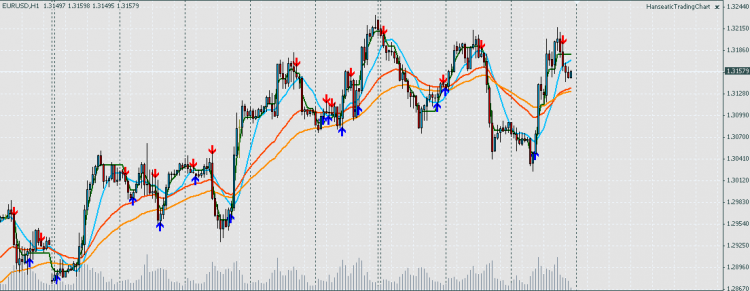# ADX Cross Indicator used in custom EA

Hello,

This is my first post. I am programming a custom EA that uses ADX Cross indicator which prints in the chart arrows to signal buys and sells. Like the following:I added another buffer to the Indicator in order to be able to read the signal, which was one of three: BUY, SELL or NOTHING.

I run the backtest on a chart and the signals where not the same as painted in the chart. The original code of the Indicator is the following:

```//+------------------------------------------------------------------+
//|                                        http://www.metaquotes.net |
//+------------------------------------------------------------------+

#property indicator_chart_window
#property indicator_buffers 2
#property indicator_color1 Blue
#property indicator_color2 Red
//---- input parameters
//---- buffers
double ExtMapBuffer1[];
double ExtMapBuffer2[];
//----
double b4plusdi, b4minusdi, nowplusdi, nowminusdi;
int    nShift;
//+------------------------------------------------------------------+
//| Custom indicator initialization function                         |
//+------------------------------------------------------------------+
int init()
{
//---- indicators
SetIndexStyle(0, DRAW_ARROW, 0, 1);
SetIndexArrow(0, 233);
SetIndexBuffer(0, ExtMapBuffer1);
//----
SetIndexStyle(1, DRAW_ARROW, 0, 1);
SetIndexArrow(1, 234);
SetIndexBuffer(1, ExtMapBuffer2);
//---- name for DataWindow and indicator subwindow label
//----
switch(Period())
{
case     1: nShift = 1;   break;
case     5: nShift = 3;   break;
case    15: nShift = 5;   break;
case    30: nShift = 10;  break;
case    60: nShift = 15;  break;
case   240: nShift = 20;  break;
case  1440: nShift = 80;  break;
case 10080: nShift = 100; break;
case 43200: nShift = 200; break;
}
//----
return(0);
}
//+------------------------------------------------------------------+
//| Custor indicator deinitialization function                       |
//+------------------------------------------------------------------+
int deinit()
{
//----
return(0);
}
//+------------------------------------------------------------------+
//| Custom indicator iteration function                              |
//+------------------------------------------------------------------+
int start()
{
int limit;
int counted_bars = IndicatorCounted();
//---- check for possible errors
if(counted_bars < 0)
return(-1);
//---- last counted bar will be recounted
if(counted_bars > 0)
counted_bars--;
limit = Bars - counted_bars;
//----
for(int i = 0; i < limit; i++)
{
//----
if(b4plusdi > b4minusdi && nowplusdi < nowminusdi)
ExtMapBuffer1[i] = Low[i] - nShift*Point;
//----
if(b4plusdi < b4minusdi && nowplusdi > nowminusdi)
ExtMapBuffer2[i] = High[i] + nShift*Point;
}
//----
return(0);
}
//+------------------------------------------------------------------+
```

My EA is executed each time a bar closes, not each tick. And the signals where different, and late. Why can that be?

Should I extract the logic from the Indicator to the EA?

Thank you

Does the Indicator re-paint ?

```for(int i = 0; i < limit; i++)
{